# Logical Reasoning for Freshers - Set 3

• In a coded message, SLOW MOVE, GET BACKWARDS, FIRE AWAY is written as VFMD ZMWE, BEN PCTLDCOXU, QHOE CDCI.
• Based on this coding scheme, spot the codes for following words
FIERCE

a. QHEHTE
b. QHEEOT
c. QHETOE
d. QHEOTE

Explanation:

 Letter G E T A W Y F I R B C K D S M O V L Code B E N C D I Q H O P T L X U Z M W F
 Letter F I E R C E Code Q H E O T E

• In a coded message, SLOW MOVE, GET BACKWARDS, FIRE AWAY is written as VFMD ZMWE, BEN PCTLDCOXU, QHOE CDCI.
• Based on this coding scheme, spot the codes for following words
BRICKS

a. POHTUL
b. POHLLU
c. POHTLU
d. POHULT

Explanation:

 Letter G E T A W Y F I R B C K D S M O V L Code B E N C D I Q H O P T L X U Z M W F
 Letter B R I C K S Code P O H T L U

3)   Choose the number which is different from others in the group.

a. 35
b. 49
c. 50
d. 63
e. 140
 Answer  Explanation ANSWER: 50 Explanation: Each number is divisible by 7, except 50.

4)   Choose the number which is different from others.

a. 7 : 343
b. 9 : 243
c. 8 : 512
d. 6 : 216
 Answer  Explanation ANSWER: 8 : 512 Explanation: In all other pairs, second number is the cube of the first number.

1. Pepper Potts, Hulk, Ironman, Hawkeye, Thor, Loki and Black Widow are sitting in a circle.
2. Pepper Potts, Hulk, Ironman, Hawkeye, Loki and Black Widow are sitting at equal distances from each other.
3. Ironman is sitting two places right of Loki, who is sitting one place right of Hawkeye.
4. Pepper Potts forms an angle of 90° from Thor and an angle of 120° from Hulk.
5. Hulk is just opposite Black Widow and is sitting on the left of Thor.

Where is Hawkeye sitting with respect to Black Widow?

a. To immediate right
b. To immediate left
c. Exactly Opposite
d. None of these
 Answer  Explanation ANSWER: To immediate right Explanation: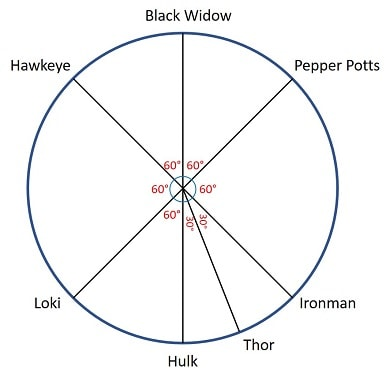Hawkeye is sitting to the immediate right of Black Widow.

1. Pepper Potts, Hulk, Ironman, Hawkeye, Thor, Loki and Black Widow are sitting in a circle.
2. Pepper Potts, Hulk, Ironman, Hawkeye, Loki and Black Widow are sitting at equal distances from each other.
3. Ironman is sitting two places right of Loki, who is sitting one place right of Hawkeye.
4. Pepper Potts forms an angle of 90° from Thor and an angle of 120° from Hulk.
5. Hulk is just opposite Black Widow and is sitting on the left of Thor.

The angle between Thor and Hulk in the clockwise direction is?

a. 150°
b. 180°
c. 210°
d. None of these
 Answer  Explanation ANSWER: None of these Explanation:The angle between Thor and Hulk in clockwise direction is 30°

7)   In the following question, various terms of an alphabet series are given with one or more missing terms as shown by (?). Choose the missing term out of the given alternatives.

U, S, P, L, G, ?

a. B
b. A
c. D
d. C
 Answer  Explanation ANSWER: A Explanation: The pattern in the given series is -2, -3, -4, -5, -6 ….U __-2 __ S __-3 __ P __-4__ L __-5__ G __-6__ ASo,the next term should be A.

8)   In the following question, various terms of an alphabet series are given with one or more missing terms as shown by (?). Choose the missing term out of the given alternatives.

L, O, J, T, H, ?

a. X
b. S
c. Z
d. W
 Answer  Explanation ANSWER: S Explanation: The given series is a combination of two series.I: L, J, HII: O, Q, ?I consists of 12th, 10th and 8th letter from the beginning of alphabets.II consists of 12th, 10th and 8th letter from the end of alphabets.So, the next letter should be 8th letter from end of alphabets i.e. S

9)   In the question below is given a statement followed by two courses of action numbered I and II. You have to assume everything in the statement to be true and based on the information given in the statement, decide which of the suggested courses of action logically follow(s) for pursuing.

(A) If only I follows
(B) If only II follows
(C) If either I or II follows
(D) If neither I nor II follow
(E) If both I and II follow.

Statement - Hundreds of eligible students fail to get admission in colleges in rural areas after passing their school examination.

Courses of Action - I. More colleges should be set up in rural areas.

II. The number of schools in rural areas should be increased.

a. Only I follows
b. Only II follows
c. None follow
d. Either I or II follow
e. Both I and II follow
 Answer  Explanation ANSWER: Only I follows Explanation: The solution to the problem lies in accommodating the increasing number of students passing out from schools. So, increasing number of schools will not solve the problem here.In order to provide better facilities to the students more colleges should be set up. So, only I follows.

10)   In the question below is given a statement followed by two courses of action numbered I and II. You have to assume everything in the statement to be true and based on the information given in the statement, decide which of the suggested courses of action logically follow(s) for pursuing.

(A) If only I follows
(B) If only II follows
(C) If either I or II follows
(D) If neither I nor II follow
(E) If both I and II follow.

Statement - Smoking duplicate cigarettes from a local shop has led to many people becoming critically ill.

Courses of Action - I. The owner of the cigarette shop should be asked to open shop elsewhere.

II. The owner of cigarette shop should immediately be arrested.

a. Only I follow
b. None follow
c. Only II follows
d. Either I or II follow
e. Neither I nor II follow
 Answer  Explanation ANSWER: Only II follows Explanation: Relocating the shop is not the solution to the problem as it may create the same problem in that place. So, II does not follow here.II follows as the criminals must be punished for selling duplicate cigarette as well as Government should conduct raids and test samples collected from different shops.

11)   In the following question, a statement/group of statements is given followed by some conclusions. Without resolving anything yourself, choose the conclusion which logically follows from the statement(s).

Statement - Many offices are located in buildings with multiple floors. If a building has more than three floors, it has a lift.

a. All floors of buildings with multiple floors may be reached in lifts.
b. Only floors above the third floor have lifts.
c. Seventh floor does not have lifts.
d. All buildings have lifts.
 Answer  Explanation ANSWER: All floors of buildings with multiple floors may be reached in lifts. Explanation: No explanation is available for this question!

12)   In the following question, a statement/group of statements is given followed by some conclusions. Without resolving anything yourself, choose the conclusion which logically follows from the statement(s).

Statement - Many creative persons become painter.

a. Some painters are creative persons.
b. A creative person will surely become painter.
c. It is not possible to become artist without creativity.
d. A high level of creativity is not needed to become a painter.
 Answer  Explanation ANSWER: Some painters are creative persons. Explanation: No explanation is available for this question!

• A billionaire drove his Ferrari towards North, then turned left and drove 10 km and again turned left and covered 20 km.
• He found himself 10 km West of his starting point.
How far did he drive northward initially?

a. 10 km
b. 15 km
c. 20 km
d. 25 km
 Answer  Explanation ANSWER: 20 km Explanation: The billionaire drove 20 km in North direction initially.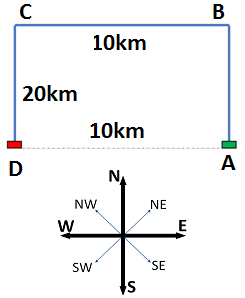14)   Study the information given below carefully, and answer the question that follow:
• On a stage, D, N, A and P are standing as described below facing North.
1) N is 2.5 m to the west of A.
2) K is 4 m to the right of A.
3) D is 6 m to the south of K.
4) P is 9 m to the north of D.

Who is to the south-west of P?

a. A
b. D
c. K
d. Neither N nor A
 Answer  Explanation ANSWER: A Explanation: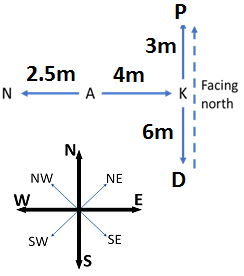A is to the south-west of P.

1. J + K means J is the son of K
2. J - K means J is the wife of K
3. J x K means J is the brother of K
4. J ÷ K means J is the mother of K
5. J = K means J is the sister of K

What does A x B ÷ C mean?

a. A is the brother of C
b. A is the father of C
c. A is the uncle of C
d. A is the nephew of C
 Answer  Explanation ANSWER: A is the uncle of C Explanation: A x B ÷ C means A is the brother of B who is the mother of C i.e. A is the uncle of C.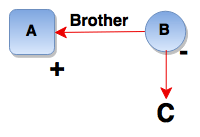16)   At what time, in minutes, between 3 o'clock and 3.25 pm, both the needles of the clock will coincide each other?

a. 13 (4/11)
b. 14 (4/11)
c. 15 (4/11)
d. 16 (4/11)
 Answer  Explanation ANSWER: 16 (4/11) Explanation: At 3 o'clock, the minute hand is 15 min. spaces apart from the hour hand.To be coincident, it must gain 15 min. spaces.55 min. are gained in 60 min15 min. spaces are gained in 60/55 x 15 = 16 4/11 minThe hands are co-incident at 16 (4/11) past 3.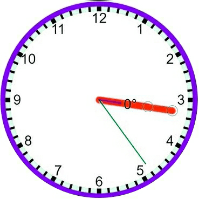17)   The question given below has a set of three or four statements. Each set of statements is further divided into three segments. Choose the alternative where the third segment in the statement can be logically deduced using both the preceding two, but not just from one of them.

Statement - A. No Z is A. All X is Z. No X is A.
B. No Z is A. All X is Z. All X is A.
C. Some Z is not A. Some X is Z. Some X is not A.

a. A
b. B
c. C
d. D
 Answer  Explanation ANSWER: A Explanation: No explanation is available for this question!

18)   In the question given below has a set of three or four statements. Each set of statements is further divided into three segments. Choose the alternative where the third segment in the statement can be logically deduced using both the preceding two, but not just from one of them.

Statement - A. All cats are mad. All sick persons are mad. So, all sick persons are cats.
B. All oranges are white. All figs are oranges. So, all figs are white.
C. No man can crawl. No kite can crawl. So, all men are kites.

a. A
b. B
c. C
d. None
 Answer  Explanation ANSWER: B Explanation: No explanation is available for this question!

19)   The following question consists of some Problem Figures followed by other figures marked 1, 2, 3, 4 called the Answer Figures.

Find out the correct answer figure that should come next in the sequence of problem figures.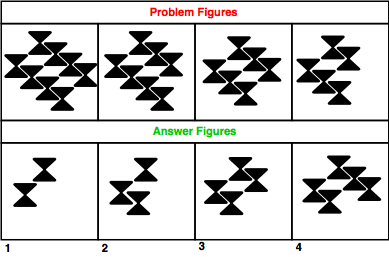a. 1
b. 2
c. 3
d. 5
 Answer  Explanation ANSWER: 3 Explanation: Elements are deleted in a set order from each row alternately. The answer figure should be figure 3.

20)   The following question consists of some Problem Figures followed by other figures marked 1, 2, 3, 4 called the Answer Figures.

Find out the correct answer figure that should come next in the sequence of problem figures.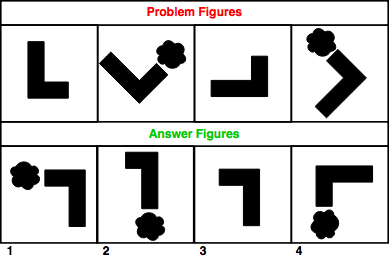a. 1
b. 2
c. 3
d. 4
 Answer  Explanation ANSWER: 3 Explanation: L-bar is rotated through 45 degree. Then, one small figure is added on the smaller side of the bar. In the next step, the bar is rotated through 45 degrees and the small figure is removed. Thus, the answer figure should be figure 3.